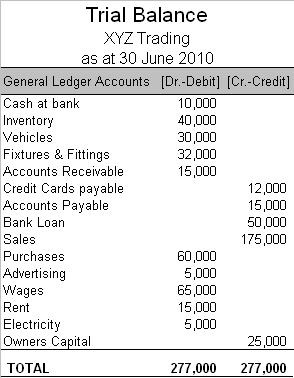# Preparation of Trial Balance

Following Steps are involved in the preparation of a Trial Balance:

• All Ledger Accounts are closed at the end of an accounting period.
• Ledger balances are posted into the trial balance.
• Trial Balance is cast and errors are identified.
• Suspense account is created to agree the trial balance totals temporarily until corrections are accounted for.
• Errors identified earlier are rectified by posting corrective entries.
• Any adjustments required at the period end not previously accounted for are incorporated into the trial balance.

### Closing Ledger Accounts

Ledger accounts are closed at the end of each accounting period by calculating the totals of debit and credit sides of a ledger. The difference between the sum of debits and credits is known as the closing balance. This is the amount which is posted in the trial balance.

How closing balances are presented in the ledger depends on whether the account is related to income statement (income and expenses) or balance sheet (assets, liabilities and equity). Balance sheet ledger accounts are closed by writing ‘Balance c/d’ next to the balancing figure since these are to be rolled forward in the next accounting period. Income statement ledger accounts on the other hand are closed by writing ‘Income Statement’ next to the residual amount because it is being transferred to the income statement as revenue or expense incurred for the period.

The steps involved in closing a ledger account may be summarized as below:

• Add the totals of both sides of a ledger
• The higher of the totals among the debit side and credit side must be inserted at the end of BOTH sides.Closing balance is the balancing figure on the side with the lower balance.
• In case of ledger accounts of assets, liabilities and equity, ‘balance c/d’ is written next to the closing balance whereas in case of income and expenses ledger accounts, ‘Income Statement’ is written next to the closing balance.
• The closing balances of all ledger accounts are posted into the trial balance.

## Trial Balance

A trial balance is a bookkeeping worksheet-like account that reflects all the credit and debit balances of all the ledger accounts. Once we prepare this statement, we can prepare the final accounts of the company on the basis of this trial balance.

One other important use of the trial balance is that it can determine to the arithmetic accuracy of the accounts. So if both columns of the trial balance tally, we can be reasonably assured of the accuracy of the accounts. It does not ensure that the accounts are free of all errors but it can at least establish mathematical accuracy.### Preparation of Trial Balance

Preparation of trial balance is the third step in the accounting process. First, we record the transactions in the journal. And then we post them in the general ledger. Then we prepare a trial balance to verify that the debit totals equal to the credit totals. Let us take a look at the steps in the preparation of trial balance.

1. To prepare a trial balance we need the closing balances of all the ledger accounts and the cash book as well as the bank book. So firstly every ledger account must be balanced. Balancing is the difference between the sum of all the debit entries and the sum of all the credit entries.
2. Then prepare a three column worksheet. One column for the account name and the corresponding columns for debit and credit balances.
3. Fill out the account name and the balance of such account in the appropriate debit or credit column
4. Then we total both the debit column and the credit column. Ideally, in a balanced error-free Trial balance these totals should be the same
5. Once you compare the totals and the totals are same you close the trial balance. If there is a difference we try and find and rectify errors. Here are some cases that cause errors in the trial balance
• A mistake in transferring the balances to the trial balance
• Error in balancing an account
• The wrong amount posted in the ledger
• Made the entry in the wrong column, debit instead of credit or vice versa
• Mistake made in the casting of the journal or subsidiary book

### Rules for Preparation of Trial Balance

While preparation of trial balances we must take care of the following rules/points

1] The balances of the following accounts are always found on the debit column of the trial balance

• Assets
• Expense Accounts
• Drawings Account
• Cash Balance
• Bank Balance
• Any losses

2] And the following balances are placed on the credit column of the trial balance

• Liabilities
• Income Accounts
• Capital Account
• Profits

## One thought on “Preparation of Trial Balance”

error: Content is protected !!